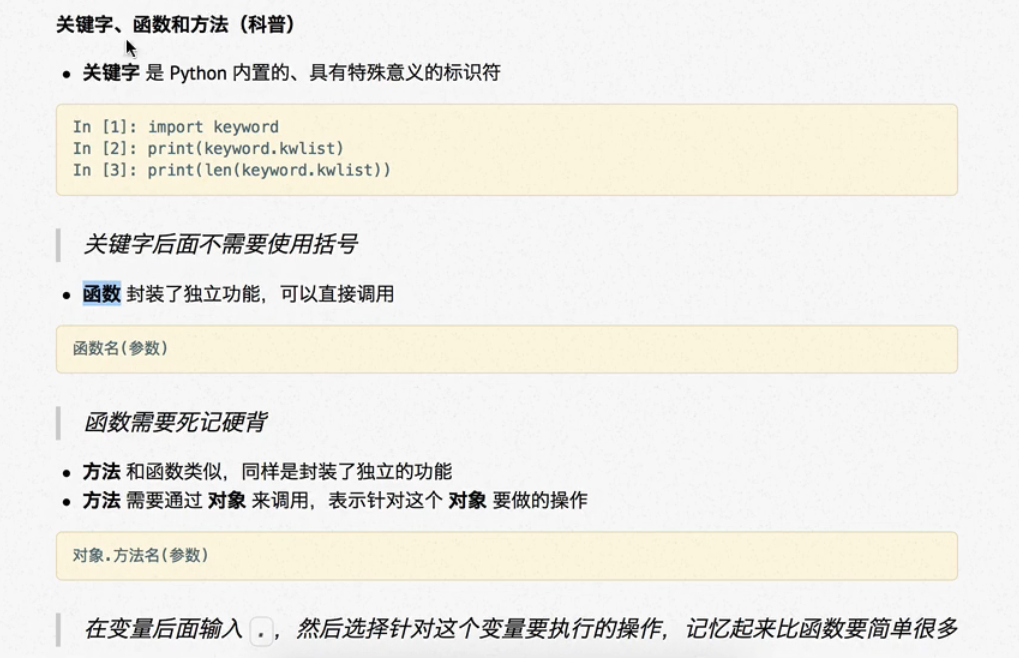本站每日ip已达10000,出租广告位,位置价格可谈,需要合作请联系站长

+关注

## python中关键字，函数，方法的理解

``````def a(i, b=[]):
b.append(i)
return b

list1 = a(10)
list2 = a(123, [])
list3 = a('a')

print(list1)
print(list2)
print(list3)
``````

[10, ‘a’]

[10, ‘a’]
#不传的话，一直用的是b，空列表

``````import keyword
print("python中关键字的个数是：%d"%len(keyword.kwlist))
print(keyword.kwlist)
# python中关键字的个数是：33
# ['False', 'None', 'True', 'and', 'as', 'assert', 'break', 'class', 'continue', 'def', 'del', 'elif', 'else', 'except', 'finally', 'for', 'from', 'global', 'if', 'import', 'in', 'is', 'lambda', 'nonlocal', 'not', 'or', 'pass', 'raise', 'return', 'try', 'while', 'with', 'yield']
``````0 0